# The Proper Axioms of the TheoryExamples

In the monograph Principia Metphysica, there are three proper axioms. The first two tell us: (1) ordinary objects, i.e., objects that possibly have a location in spacetime, necessarily do not encode properties, and (2) objects x and y bear the E-identity relation iff x and y are both ordinary objects and (necessarily) they exemplify the same properties. These two axioms may be formally represented as follows: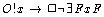The third proper axiom is a comprehension principle that asserts conditions under which abstract objects exist: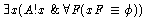We complete this tutorial with 4 example sentences that are instances of this axiom schema. Of course, there are a denumerably infinite number of other sentences which are axioms as well.

## Examples of the Comprehension Axiom for Abstract Objects

1) There is an abstract object that encodes all and only the properties Clinton exemplifies.If we suppose that the constant symbol `c ' denotes Bill Clinton, then sentence is instance of the comprehension principle which asserts that there exists an abstract object which encodes exactly the properties that Clinton exemplifies. This is a perfect, abstract model of Clinton. If we use Leibniz's terminology, we might say that this object is an individual concept that contains all the general concepts that apply to Clinton. In other words, this is Clinton's monad. There are a lot of interesting theorems that govern monads, and these are described in the section of Principia Metaphysica entitled The Theory of Monads.

2) There is an abstract object that encodes all and only the properties that Holmes exemplifies in the story s.Let us assume for the moment that the phrase `In the story s' is well-defined. That is, let us assume: (1) that there is a story s which is the classical source of information about Sherlock Holmes (we may think of this as the conjunction of Conan Doyle stories about Holmes, conceived as one lengthy story), and that (2) there is some determinate set of properties F such that the proposition Holmes exemplifies F is true in the story s. At the very least, we take as data that there is a set of ordinary English sentences of the form `According to the story s, Holmes is F ' which are true. Then the above instance of the comprehension axiom for abstract objects asserts that there is an abstract object that encodes just those properties F that satisfy these sentences. Let us assume further that there is a unique object that encodes just those properties (this is true and can be proven). Then we claim that Sherlock Holmes just is this abstract object. Holmes encodes the properties attributed to him in the story, and so is an incomplete object (with respect to the properties he encodes). That is, there are properties F such that neither F nor the negation of F are attributed to Holmes in the story. However, like every object whatsoever, no matter whether ordinary or abstract, Holmes is complete with respect to the properties he exemplifies. For every property F, either Holmes exemplifies F or exemplifies the negation of F. He exemplifies such properties as not being a detective, not living in London, not being Watson's friend, etc., because he is an abstract object and abstract objects do not exemplify such ordinary properties. However, Holmes will exemplify such properties as being admired by some real detectives, being conceived by Conan Doyle, being famous among devotees of detective novels, etc.

3) There is an abstract object that encodes just the two properties being round and being square.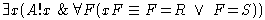Let `R ' denote the property of being round and `S ' denote the property of being square. Then this instance of comprehension asserts that there is an abstract object that encodes both of those properties and no others. Why is it that a disjunction (`or') is used in the right side of the biconditional rather than a conjunction (`and')? Well, consider the following description of a set: {x | x=1 V x=2}. How many elements does this set have? The answer is: two. It describes the set {1,2}, for only the numbers 1 and 2 satisfy the defining condition `x=1 V x=2'. Similarly, the set {F | F=R V F=S} is a description of the set containing both the properties of being round and being square and no others; i.e., {R,S}. So if we take the condition `F=R V F=S' and use it as the right condition of the biconditional in an instance of the comprehension axiom, we assert the existence of an abstract object encoding two properties. NOTE: This abstract object is not inconsistent with the law of geometry that says: necessarily everything round fails to be square. This law is a law that governs objects that exemplify roundness, not those that encode it. It is rendered into formal notation as: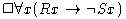The present instance of comprehension merely asserts that there is an abstract object that encodes roundness and squareness; it is logically compatible with the above law of geometry for it does not follow from the fact that an object encodes a property that it also exemplifies that property.

4) There is an abstract object that encodes just the propositional properties F that are constructed out of true propositions.We say that a property F is a propositional property constructed out of proposition p just in case F just is the property being such that p. The property being such that p is represented by the following predicate: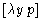An object x exemplifies this property iff p (is true). So being such that p is a propositional property constructed out of a true proposition whenevern p is true. Our instance of comprehension asserts that there is an abstract object that encodes all and only such properties. In the section entitled The Theory of Worlds, this object will provably satisfy the definition of an `actual world'. We shall extend our notion of encoding so that we may say that an object x encodes proposition p whenever x encodes the property being such that p. So the above object encodes all and only true propositions: it encodes all (and only) that (which) is the case.